### Why should I choose AnalystNotes?

AnalystNotes specializes in helping candidates pass. Period.

##### Subject 8. Hypothesis Tests Concerning the Mean: T-Test vs. Z-Test
When testing a hypothesis concerning the value of a population mean, either a t-test or a z-test can be conducted.

• A t-test is a hypothesis test that uses a t-statistic, which follows a t-distribution.
• A z-test is a hypothesis that uses a z-statistic, which follows a z-distribution (a standard normal distribution).

Deciding when to use a t-test or a z-test depends on three factors:

• Distribution of population: normal or non-normal
• Population variance: known or unknown
• Sample size: large or small

When to use the t-test?

This test should be used if the population variance is unknown and either of the following conditions holds:

• The sample size is large (in general, n ≥ 30).
• The sample size is small (n ≤ 30) but the population is normally distributed or approximately normally distributed.

For a t-test concerning a single population mean (μ), the test statistic to be used is the t-statistic with n - 1 degrees of freedom.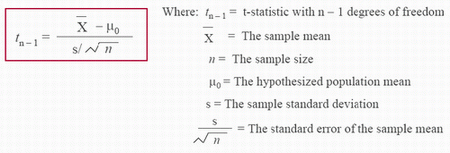How to make the decision to conduct a t-test?

• Calculate the t-statistic with n - 1 degrees of freedom.

• Use a t-table to find the rejection point(s) at the specified level of significance with n - 1 degrees of freedom.

A portion of a t-table is presented below: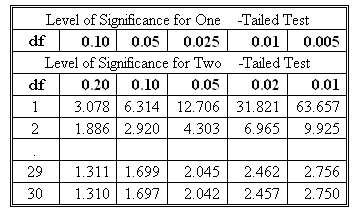Note: This t-table is a combination of a one-tailed and a two-tailed table. On the exam, you may be asked to conduct a two-tailed hypothesis test using a one-tailed table or a one-tailed test using a two-tailed table.

• Compare the calculated value of the t-statistic with the rejection point(s) to make the decision.

• For a two-tailed test (H0: μ = μ0 versus Ha: μ ≠ μ0), there are two rejection points. Reject the null if the t-statistic is greater than the upper rejection point or less than the lower rejection point.
• For a one-tailed test (H0: μ ≤ μ0 versus Ha: μ > μ0), there is only one rejection point: the upper rejection point. Reject the null if the t-statistic is greater than the upper rejection point.
• For a one-tailed test (H0: μ ≥ μ0 versus Ha: μ < μ0), there is only one rejection point: the lower rejection point. Reject the null if the t-statistic is less than the upper rejection point.

When to use the z-test?

The z-test should be used if the population variance is normally distributed with known variance.

For a z-test concerning a single population mean (μ), the test statistic to be used is the z-statistic with n degrees of freedom.To conduct a z-test, compare the calculated value of the z-statistic with rejection point(s) at the specified level of significance. Rejection points are obtained using a z-table.

Most Frequently Used Rejection Points for a z-test:For a population with unknown variance, it is acceptable to use the z-test if the sample size is large.

• The t-test should be used if the population variance is unknown and the sample size is large.
• In this case, it is also acceptable to use the z-test because of the central limit theorem. Recall that according to the central limit theorem, if the sample size is sufficient large, the sampling distribution of the sample mean will be approximately normally distributed.
• The z-statistic is computed below:If you are interested, there is a detailed explanation of this last point at the end of this subject (the z-test and the central limit theorem).

Summary

• In practice, the population variance is typically unknown.
• The table below summarizes tests concerning the population mean when the population has unknown variance.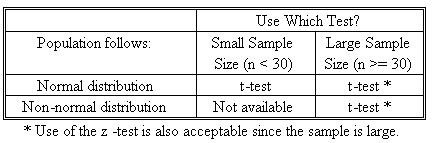Example

The Jones Fund has been in existence for 20 years. Monthly returns over this period are approximately normally distributed. A random sample of 20 monthly returns shows that the fund has achieved a mean monthly return of 2%. The sample standard deviation of monthly returns is 3%. The Jones Fund was expected to have earned a 1.5% mean monthly return over the 20-year period. Using a 5% significance level, determine if the fund's actual performance is consistent with the expected mean monthly return of 1.5%.

Solution

1. State the hypothesis. Let μ be the mean monthly return on the Jones Fund. The null and alternative hypotheses are stated as:

• H0: μ = μ0 (The mean monthly return is 1.5%.)
• Ha: μ ≠ μ0 (The mean monthly return is not 1.5%.)

Note that this is a two-tailed test.

2. Identify the test statistic. The t-statistic should be used because the population variance is unknown, the sample is small (less than 30) and the population is approximately normally distributed.

3. Specify the level of significance (α); α is given at 5%.

4. State the decision rule. You need to find the two rejection points for this two-tailed test in a t-table.

A portion of a one-tailed t-table is given below: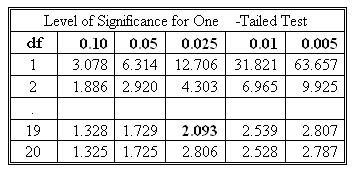• The degree of freedom = n - 1 = 19.
• Since this is a two-tailed test and you are given a one-tailed t-table, we need to divide the 5% level of significance by 2: 0.05/2 = 0.025.
• In the one-tailed t-table, find the 19 df row and then move to the 0.025 column. The entry of 2.093 is the right-tail rejection point. Since the t-distribution is symmetrical, the left-tail rejection point is -2.093.
• Thus, the decision rule is stated as reject H0 if t < -2.093 or t > 2.093.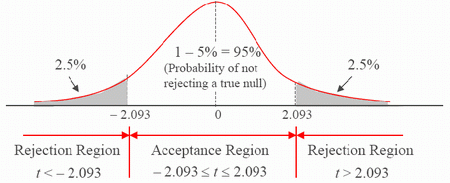5. Compute the test statistic: t19 = (0.02 - 0.015) / [0.03 / 201/2] = 0.745.

6. Make the statistical decision. Because 0.745 does not satisfy either t < -2.093 or t > 2.093, you do not reject the null hypothesis.

Thus, it is reasonable to believe that the actual performance of the Jones Fund is consistent with the expected mean monthly return of 1.5%.

The z-test and the central limit theorem.

When hypothesis testing a population mean, there are generally two options for the test statistic:

• tn-1 = (x-bar - μ0)/(s/n1/2): when the population variance is unknown and must be estimated from the sample.
• z = (x-bar - μ0)/(σ/n1/2): when the population variance is known.

The first statistic may be used if either the sample is large (n = 30 or greater) or, if n < 30, it may be used if the sample is at least approximately normally distributed. In most cases, this will be the statistic used, because in most practical problems, the population variance is not known with certainty.

The second statistic is sometimes used with large sample sizes, because the central limit theorem implies that the distribution of a sample mean will be approximately normally distributed as the sample size increases.

Recall that the degrees of freedom in a t-distribution depend on sample size and are generally defined as n-1. This means that as the sample size increases, so the degrees of freedom increase, and a t-graph begins to resemble a z-graph.

In fact, for infinity degrees of freedom (a theoretical concept, because it is not possible to have an infinite sample size), the two graphs are identical, and critical values for a z-distribution can also be found on a t-table in the row that has infinity as its degrees of freedom.

What actually happens is that, as the degrees of freedom increase, the tails of a t-graph flatten out; the graph becomes more peaked in the center and its standard deviation approaches 1 from above. The graph thus begins to resemble a z-graph in all aspects.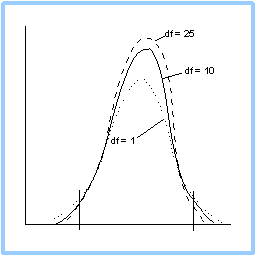As can be seen above, when the degrees of freedom are low, the graph is fairly flat in the center and has long tails and a bigger standard deviation. As the degrees of freedom increase, the tails become narrower and flatter and the graph peaks in the center.

Recall also that the area under any graph is 1, as this area represents a probability. So, as the degrees of freedom increase, the area that is "lost" in the flatter tails is "found" in the center of the graph, and that is why the graph becomes more peaked. However,

• There are differences between the t-test critical values and the z-test critical values (these can be significant but get smaller with large samples).
• The t-test is still the theoretically proper choice unless the population variance is known.

Learning Outcome Statements

g. identify the appropriate test statistic and interpret the results for a hypothesis test concerning the population mean of both large and small samples when the population is normally or approximately distributed and the variance is 1) known or 2) unknown;

CFA® 2020 Level I Curriculum, 2020, Volume 1, Reading 11

User Comment
GeoffT variance known > z-test (nozy)
variance unknown> t-test
danlan How can I get Student t-distribution table on Texas BA calculator or HP Financial Calculator,
for example the value of t(0.025, 4) ...
fding Hi Danlan:

Are you sure that the calculator? I checked its GuideBook but did NOT find such a function.
zrar the calculator has not the function,you have to do it by yourself!
achu t-test is the 'most' correct choice whenever pop variance is unknown (and being est'd by sample var).
julescruis great example
taysys1 You will have a table
johntan1979 With lots of good food on it
FozzeyBear johntan1979 is a legend, he comments on everything
dbedford So in prior LoS examples etc... Ho is what you want to reject to make your hypothesis true. In the above example the Hypothesis is that the expected return is 1.5 so you would think then that Ho would be to reject anything that says the mean is NOT 1.5, why did they make Ho M = Mo instead of M=/= Mo
dbedford ok so after further review: the Ho is the place where nothing new is happening H1 is where something new is happening. So if the expected return was 1.5 and we want to see if nothing changed about that 1.5 then Ho: M = Mo if we were to say that the expected return was 1.5 and we thought that it should be something different then H1 is M=Mo
dbedford sorry I meant that H1 is M=/=Mo. Just keep it simple Ho is where nothing new is happening H1 is where something new is happening